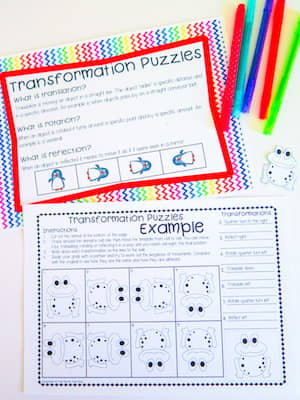# How To Make Mental Math Strategies Easier

Mental math can be challenging—and so can learning the strategies. Today I discuss how to make 5 mental math strategies easier.Despite computers and calculators and even scratch paper, we do some estimating and calculating in our heads. In fact, students need to know mental math. It helps them build a sense of numbers and how they work. It also increases accuracy and gives a solid foundation for other math. On top of that, it’s just plain practical. Mental math helps with things like figuring out a tip, determining price in a 20% off sale, or estimating the size of a room.

We often think of easy math as “math you can do in your head,” but mental math can be challenging—and so can learning the strategies. Let’s make mental math a little easier.

## Make 5 mental math strategies easier

Five essential mental math strategies are:

• Counting on
• Near doubles
• Compatible numbers
• Partitioning
• Estimating strategy

Let’s take a look at each of them.

### Counting on

When you are adding two numbers, you “count on” from one. Students need to learn to count on from the larger number of the two numbers because it is much more efficient.

For example, if you were adding 2 + 8, you would start with 8 and “count on” two. 8 – 9 – 10

Students might be tempted to start with the 2 here since it comes first. To get past this, have them first circle the larger number and then count on.

Students can use counters, their fingers or a number line/track to count on as they practice this strategy. Eventually, they should move beyond needing the counting tools.### Near doubles

If your students have learned how to double numbers, they are ready for the near doubles strategy. This strategy is used to add two consecutive numbers.

If children know that 4 + 4 = 8, they can use that knowledge to figure out what 4 + 5 equals. This is the same as 4 + 4 + 1 = 9.

You can use counters to demonstrate near doubles. Have students count out two groups of 4 counters. Then have them add a group of 1. Have them count all the counters. Then have them move the 1 group into one of the 4 groups (making groups of 4 and 5). Have them count the counters again.

Then ask students to try with another set of numbers, 6 + 7 for example. Ask: What is 6 doubled? After they answer 12, ask: Now what is 6 + 7? If they don’t get 13, have the counters or counting on to get the answer and see the pattern of double and near double.### Compatible numbers

Compatible numbers are numbers that when added produce a “tidy sum,” one that usually ends in zero, or multiples of 10.

Examples include 6 and 4; 8 and 2; and 9 and 1. When added, these number pairs add up to 10 (end in zero). Other examples include 40 and 60; 17 and 83; and 32 and 68, because when these numbers are added they total 100 (end in zero).

Use numbers strips of 10 and ask students to show different compatible number sets that add up to 10. Or give each student a number 0 – 10 and have them find a partner with a compatible number.### Partitioning

When we use the partitioning strategy, we break numbers into parts to simplify math. For example, take 9 + 5. If you know that 9 + 1 = 10, you use partitioning. You partition the 5 into a 1 and a 4. You first add 9 and 1 to total 10, and then add on the 4.

While using partitioning in combination with compatible numbers is common, you can partition numbers any way that helps you solve the problem. For example, 26 + 7 could be partitioned as

20 + (6 + 7) = 20 + 13 = 33

(26 + 4) + 3 = 30 + 3 = 33

The first example uses partitioning plus near doubles strategy. The second uses partitioning and compatible numbers.

You can use partitioning for subtraction, multiplication, and division as well as addition.### Estimating

Estimating is an important strategy because it allows us to judge the reasonableness of  responses. Sometimes when students are struggling with math or doing their work too quickly they come up with an answer that is way off. Estimating can help them asses whether their answer is reasonable.

Rounding, finding compatible numbers, and using benchmarks are three techniques students can use in estimating. Take the classic guess how many candies in a jar game. You could just guess, or you could try to estimate by benchmarking. Look at the bottom of the jar. How many candies can you see? Now look at about how many layers of candy there are. Use those two numbers to estimate how many candies are in the jar as a whole.

Mental math strategies are developed with lots of practice. Math activities and games keep this practice fun.

My Mental Math Activities and Posters make it easy on you. You get:

• An explanation of each strategy with examples;
• Posters to assist in explaining each strategy;
• Games to provide further consolidation of the strategy.

Make teaching mental math strategies easier, your students can get the practice they need on these essential skills. All the details are here:

Mental Math Activities and Posters### FREE Math Games & Activities